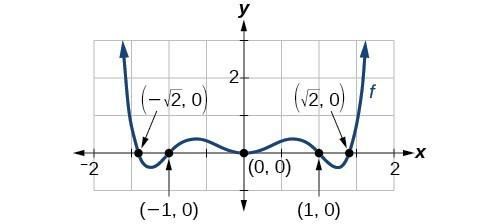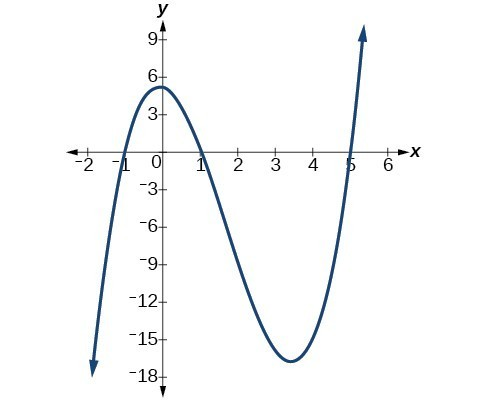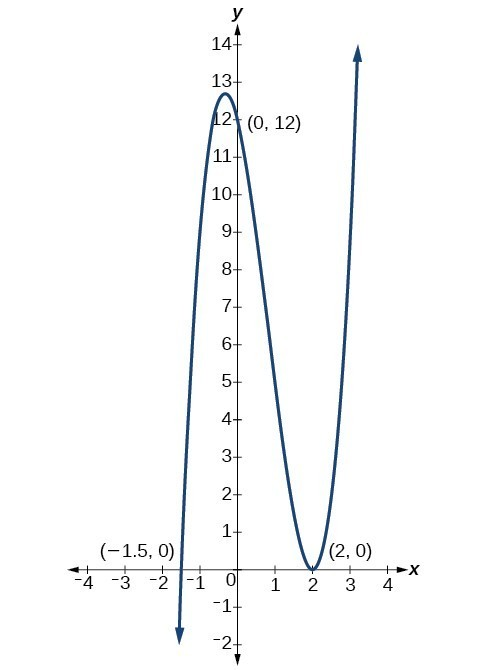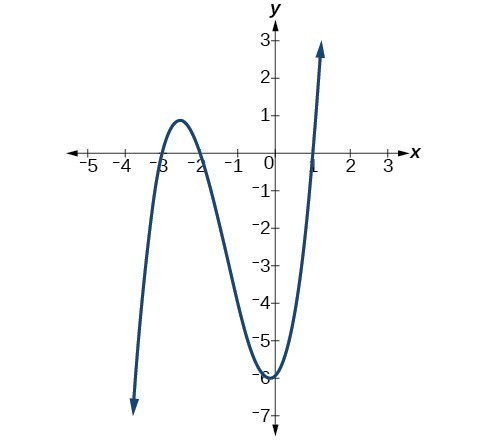## Use factoring to ﬁnd zeros of polynomial functions

Recall that if f is a polynomial function, the values of x for which $f\left(x\right)=0$ are called zeros of f. If the equation of the polynomial function can be factored, we can set each factor equal to zero and solve for the zeros.

We can use the method of factoring the polynomial function and setting each factor equal to zero to find x-intercepts because at the x-intercepts we find the input values when the output value is zero. For general polynomials, this can be a challenging prospect. While quadratics can be solved using the relatively simple quadratic formula, the corresponding formulas for cubic and fourth-degree polynomials are not simple enough to remember, and formulas do not exist for general higher-degree polynomials. Consequently, we will limit ourselves to three cases in this section:

1. The polynomial can be factored using known methods: greatest common factor and trinomial factoring.
2. The polynomial is given in factored form.
3. Technology is used to determine the intercepts.

### How To: Given a polynomial function f, find the x-intercepts by factoring.

1. Set $f\left(x\right)=0$.
2. If the polynomial function is not given in factored form:
1. Factor out any common monomial factors.
2. Factor any factorable binomials or trinomials.
3. Set each factor equal to zero and solve to find the $x\text{-}$ intercepts.

### Example 2: Finding the x-Intercepts of a Polynomial Function by Factoring

Find the x-intercepts of $f\left(x\right)={x}^{6}-3{x}^{4}+2{x}^{2}$.

We can attempt to factor this polynomial to find solutions for $f\left(x\right)=0$.

$\begin{cases}{x}^{6}-3{x}^{4}+2{x}^{2}=0 \hfill & \text{Factor out the greatest common factor}. \hfill \\ \text{ }{x}^{2}\left({x}^{4}-3{x}^{2}+2\right)=0\hfill & \text{Factor the trinomial}.\hfill \\ {x}^{2}\left({x}^{2}-1\right)\left({x}^{2}-2\right)=0\hfill & \text{Set each factor equal to zero}.\hfill \end{cases}$
$\begin{cases}\hfill & \hfill & \left({x}^{2}-1\right)=0\hfill & \hfill & \left({x}^{2}-2\right)=0 \\ {x}^{2}=0 \hfill & \text{or}\hfill &{x}^{2}=1\hfill & \text{or}\hfill &{x}^{2}=2 \\ x=0\hfill & \hfill & x=\pm 1\hfill & \hfill & x=\pm \sqrt{2}\hfill \end{cases}$Figure 3

This gives us five x-intercepts: $\left(0,0\right),\left(1,0\right),\left(-1,0\right),\left(\sqrt{2},0\right)$, and $\left(-\sqrt{2},0\right)$. We can see that this is an even function.

### Example 3: Finding the x-Intercepts of a Polynomial Function by Factoring

Find the x-intercepts of $f\left(x\right)={x}^{3}-5{x}^{2}-x+5$.

### Solution

Find solutions for $f\left(x\right)=0$ by factoring.

$\begin{cases} \text{ }{x}^{3}-5{x}^{2}-x+5=0\hfill & \text{Factor by grouping}.\hfill \hfill \\ \text{ }{x}^{2}\left(x - 5\right)-\left(x - 5\right)=0\hfill & \text{Factor out the common factor}.\hfill \\ \text{ }\left({x}^{2}-1\right)\left(x - 5\right)=0\hfill & \text{Factor the difference of squares}.\hfill \\ \left(x+1\right)\left(x - 1\right)\left(x - 5\right)=0\hfill & \text{Set each factor equal to zero}.\hfill \end{cases}$
$\begin{cases}x+1=0\hfill & \text{or}\hfill & x - 1=0\hfill & \text{or}\hfill & x - 5=0\hfill \\ x=-1\hfill & \hfill & x=1\hfill & \hfill & x=5\hfill \end{cases}$Figure 4

There are three x-intercepts: $\left(-1,0\right),\left(1,0\right)$, and $\left(5,0\right)$.

### Example 4: Finding the y– and x-Intercepts of a Polynomial in Factored Form

Find the y– and x-intercepts of $g\left(x\right)={\left(x - 2\right)}^{2}\left(2x+3\right)$.

### Solution

The y-intercept can be found by evaluating $g\left(0\right)$.

$\begin{cases}g\left(0\right)={\left(0 - 2\right)}^{2}\left(2\left(0\right)+3\right)\\ =12\end{cases}$

So the y-intercept is $\left(0,12\right)$.

The x-intercepts can be found by solving $g\left(x\right)=0$.

${\left(x - 2\right)}^{2}\left(2x+3\right)=0$
$\begin{cases}{\left(x - 2\right)}^{2}=0\hfill & \hfill & \hfill & \hfill & \left(2x+3\right)=0\hfill \\ \text{ }x - 2=0\hfill & \hfill & \text{or}\hfill & \hfill & \text{ }x=-\frac{3}{2}\hfill \\ \text{ }x=2\hfill & \hfill & \hfill & \hfill & \hfill \end{cases}$

So the x-intercepts are $\left(2,0\right)$ and $\left(-\frac{3}{2},0\right)$.

### Analysis of the Solution

We can always check that our answers are reasonable by using a graphing calculator to graph the polynomial as shown in Figure 5.Figure 5

### Example 5: Finding the x-Intercepts of a Polynomial Function Using a Graph

Find the x-intercepts of $h\left(x\right)={x}^{3}+4{x}^{2}+x - 6$.

### Solution

This polynomial is not in factored form, has no common factors, and does not appear to be factorable using techniques previously discussed. Fortunately, we can use technology to find the intercepts. Keep in mind that some values make graphing difficult by hand. In these cases, we can take advantage of graphing utilities.

Looking at the graph of this function, as shown in Figure 6, it appears that there are x-intercepts at $x=-3,-2$, and 1.Figure 6

We can check whether these are correct by substituting these values for x and verifying that

$h\left(-3\right)=h\left(-2\right)=h\left(1\right)=0$.

Since $h\left(x\right)={x}^{3}+4{x}^{2}+x - 6$, we have:

$\begin{cases}h\left(-3\right)={\left(-3\right)}^{3}+4{\left(-3\right)}^{2}+\left(-3\right)-6=-27+36 - 3-6=0\hfill \\ h\left(-2\right)={\left(-2\right)}^{3}+4{\left(-2\right)}^{2}+\left(-2\right)-6=-8+16 - 2-6=0\hfill \\ \text{ }h\left(1\right)={\left(1\right)}^{3}+4{\left(1\right)}^{2}+\left(1\right)-6=1+4+1 - 6=0\hfill \end{cases}$

Each x-intercept corresponds to a zero of the polynomial function and each zero yields a factor, so we can now write the polynomial in factored form.

$\begin{cases}h\left(x\right)={x}^{3}+4{x}^{2}+x - 6\hfill\hfill \\ \text{ }=\left(x+3\right)\left(x+2\right)\left(x - 1\right)\hfill \end{cases}$

### Try It 1

Find the y– and x-intercepts of the function $f\left(x\right)={x}^{4}-19{x}^{2}+30x$.

Solution